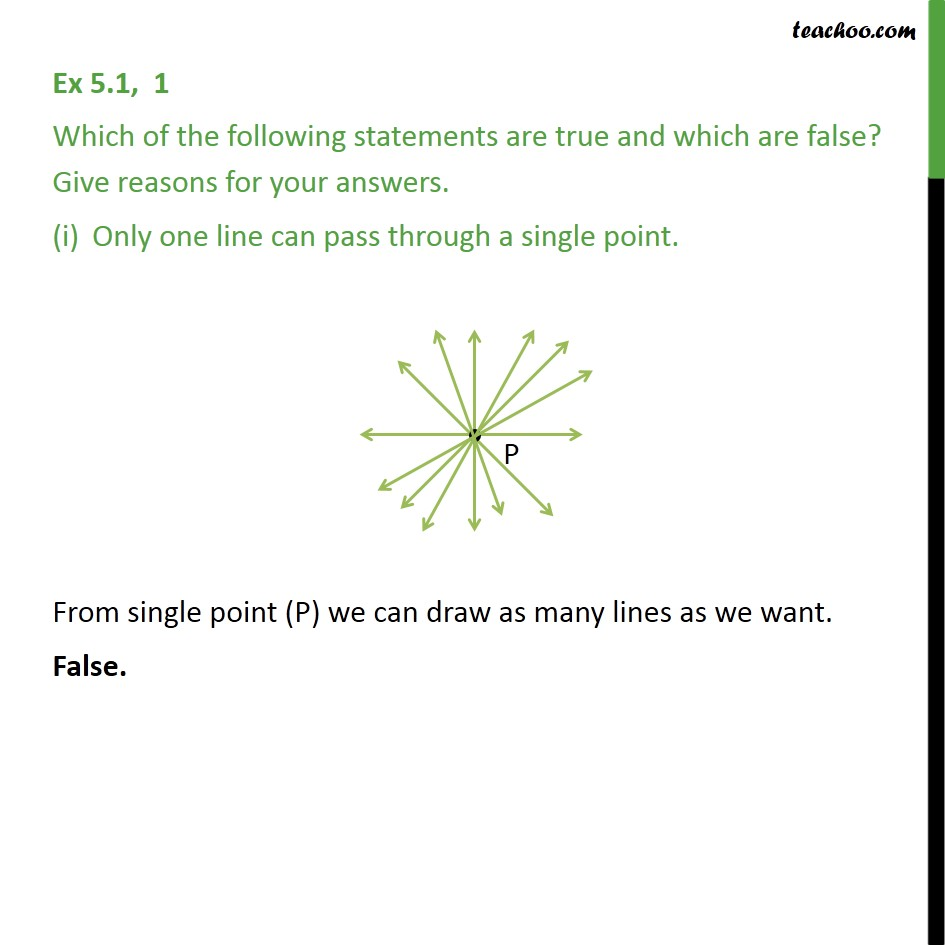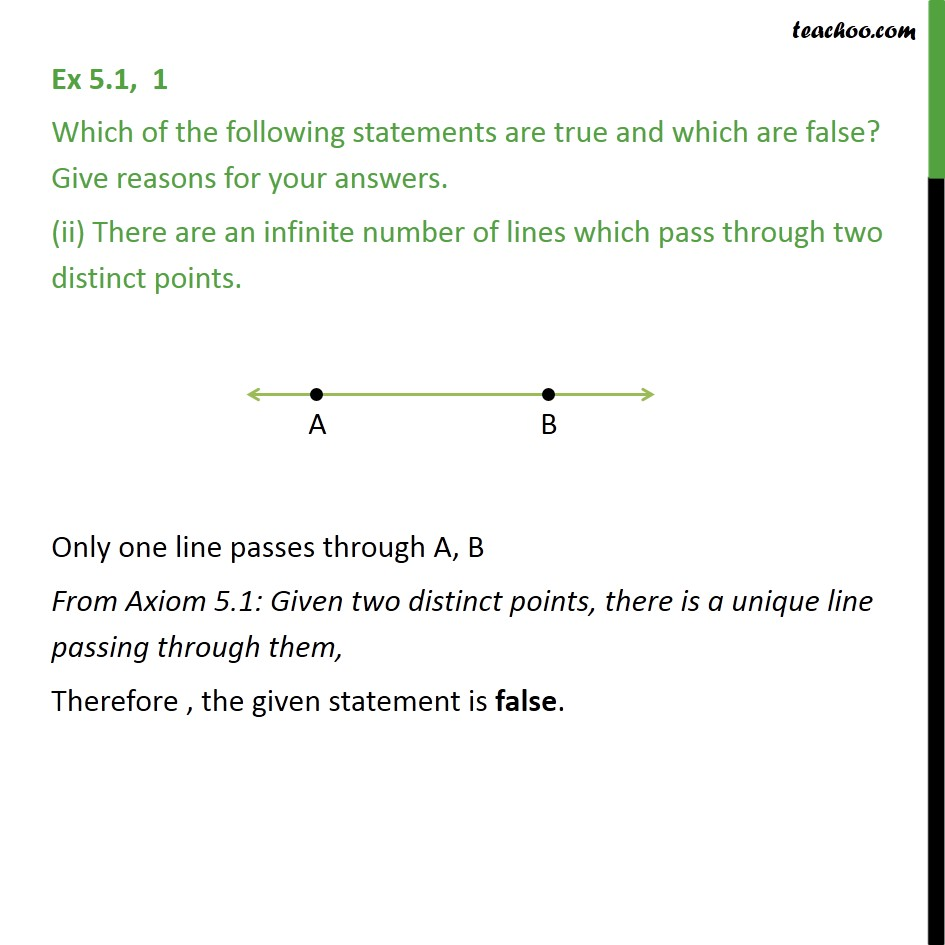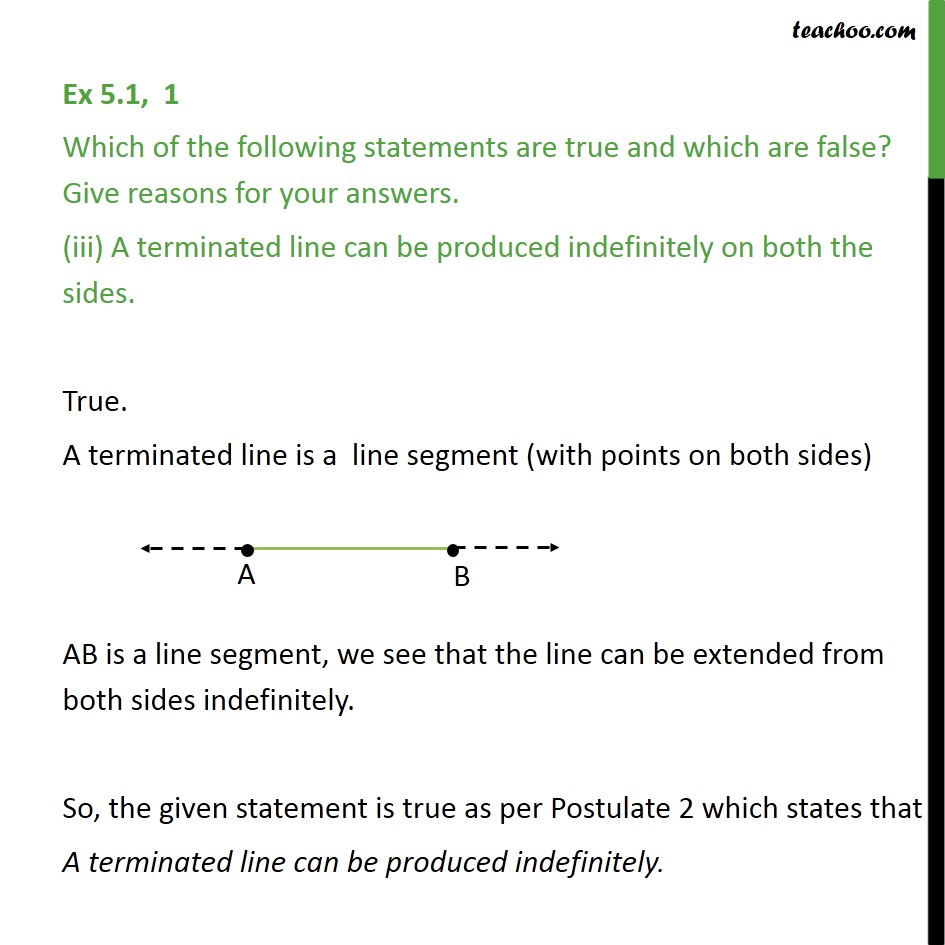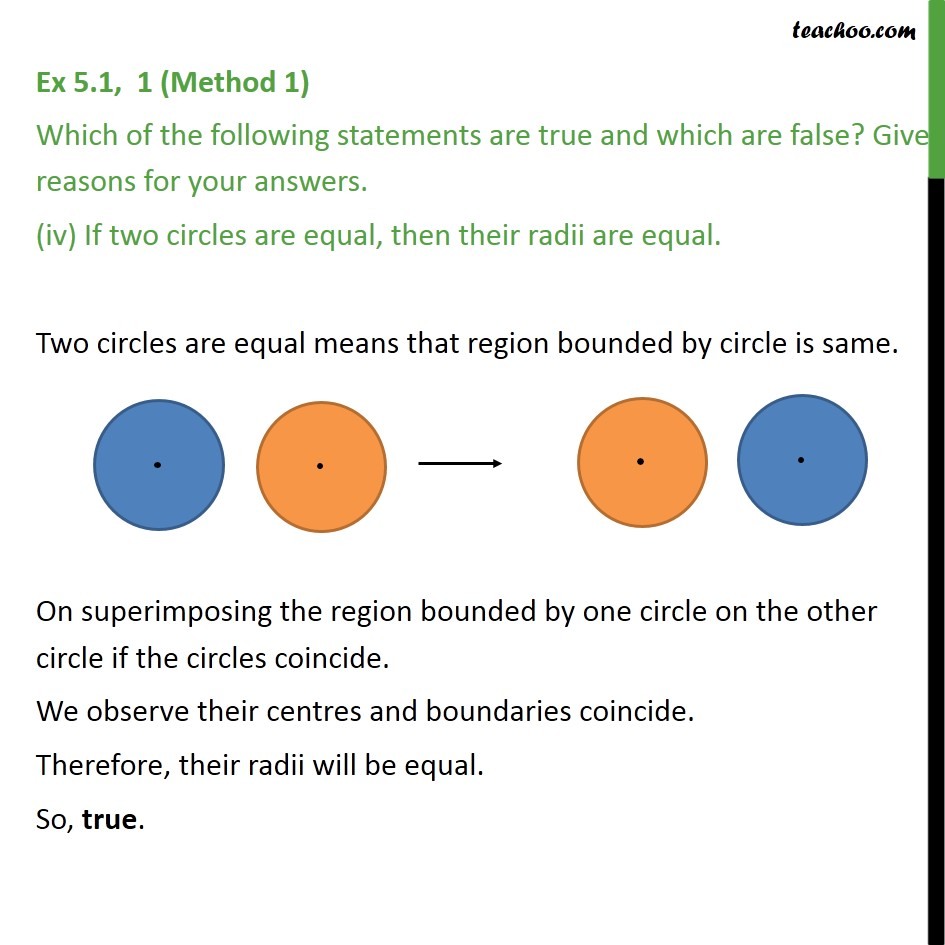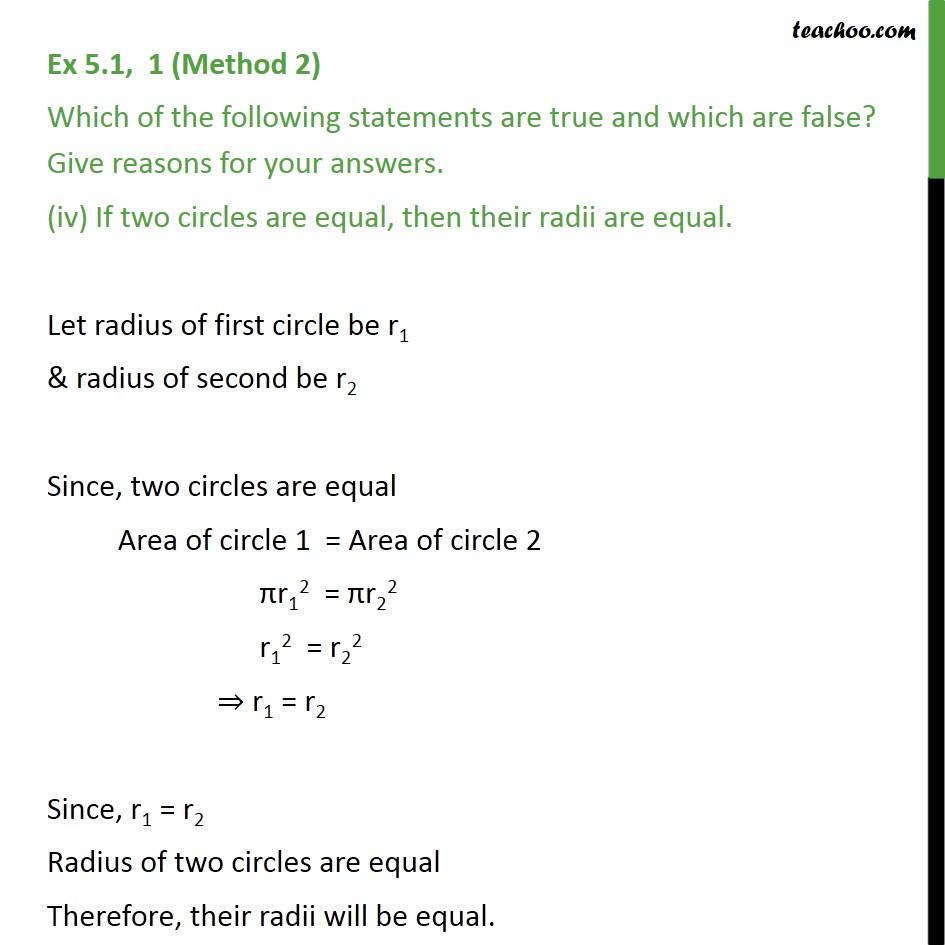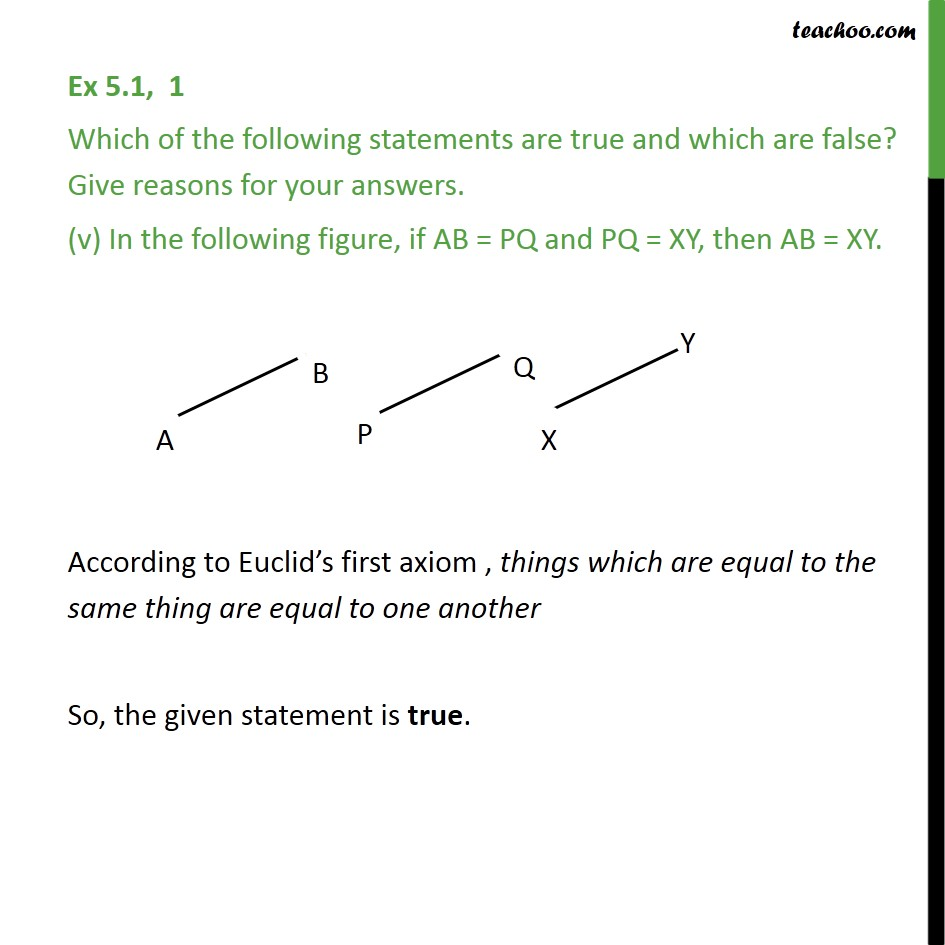1. Chapter 5 Class 9 Introduction to Euclid's Geometry
2. Serial order wise
3. Ex 5.1

Transcript

Ex 5.1, 1 Which of the following statements are true and which are false? Give reasons for your answers. Only one line can pass through a single point. From single point (P) we can draw as many lines as we want. False. Ex 5.1, 1 Which of the following statements are true and which are false? Give reasons for your answers. (ii) There are an infinite number of lines which pass through two distinct points. Only one line passes through A, B From Axiom 5.1: Given two distinct points, there is a unique line passing through them, Therefore , the given statement is false. Ex 5.1, 1 Which of the following statements are true and which are false? Give reasons for your answers. (iii) A terminated line can be produced indefinitely on both the sides. True. A terminated line is a line segment (with points on both sides) AB is a line segment, we see that the line can be extended from both sides indefinitely. So, the given statement is true as per Postulate 2 which states that A terminated line can be produced indefinitely. Ex 5.1, 1 (Method 1) Which of the following statements are true and which are false? Give reasons for your answers. (iv) If two circles are equal, then their radii are equal. Two circles are equal means that region bounded by circle is same. On superimposing the region bounded by one circle on the other circle if the circles coincide. We observe their centres and boundaries coincide. Therefore, their radii will be equal. So, true. Ex 5.1, 1 (Method 2) Which of the following statements are true and which are false? Give reasons for your answers. (iv) If two circles are equal, then their radii are equal. Let radius of first circle be r1 & radius of second be r2 Since, two circles are equal Area of circle 1 = Area of circle 2 πr12 = πr22 r12 = r22 ⇒ r1 = r2 Since, r1 = r2 Radius of two circles are equal Therefore, their radii will be equal. Ex 5.1, 1 Which of the following statements are true and which are false? Give reasons for your answers. (v) In the following figure, if AB = PQ and PQ = XY, then AB = XY. According to Euclid’s first axiom , things which are equal to the same thing are equal to one another So, the given statement is true.

Ex 5.1

About the AuthorDavneet Singh
Davneet Singh is a graduate from Indian Institute of Technology, Kanpur. He has been teaching from the past 9 years. He provides courses for Maths and Science at Teachoo.